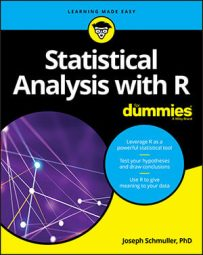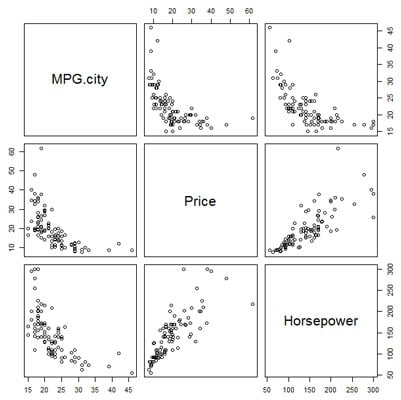##### Statistical Analysis with R For DummiesBase R provides a nice way of visualizing relationships among more than two variables. If you add price into the mix and you want to show all the pairwise relationships among MPG-city, price, and horsepower, you'd need multiple scatter plots. R can plot them all together in a matrix, as the figure shows.Multiple scatter plots for the relationships among MPG-city, price, and horsepower.

The names of the variables are in the cells of the main diagonal. Each off-diagonal cell shows the scatter plot for its row variable (on the y-axis) and its column variable (on the x-axis). For example, the scatter plot in the first row, second column shows MPG-city on the y-axis and price on the x-axis. In the second row, first column, the axes are reversed: MPG city is on the x-axis, and price is on the y-axis.

The R function for plotting this matrix is `pairs()`. To calculate the coordinates for all scatter plots, this function works with numerical columns from a matrix or a data frame.

For convenience, you create a data frame that's a subset of the `Cars93` data frame. This new data frame consists of just the three variables to plot. The function `subset()` handles that nicely:

```cars.subset <- subset(Cars93, select = c(MPG. city,Price,Horsepower)) ``` The second argument to subset creates a vector of exactly what to select out of `Cars93`. Just to make sure the new data frame is the way you want it, use the `head() `function to take a look at the first six rows:

`> head(cars.subset)`

`MPG.city Price Horsepower`

```1 25 15.9 140 ``` ```2 18 33.9 200 ``` ```3 20 29.1 172 ``` ```4 19 37.7 172 ``` ```5 22 30.0 208 ``` ```6 22 15.7 110 ``` And now,

```> pairs(cars.subset) ``` creates the plot shown.

This capability isn't limited to three variables, nor to continuous ones. To see what happens with a different type of variable, add `Cylinders `to the vector for select and then use the `pairs() `function on `cars.subset`.

## Box plots

To draw a box plot, you use a formula to show that `Horsepower `is the dependent variable and `Cylinders `is the independent variable:

`> boxplot(Cars93\$Horsepower ~ Cars93\$Cylinders, xlab="Cylinders", ylab="Horsepower")`

If you get tired of typing the \$-signs, here's another way:

```> boxplot(Horsepower ~ Cylinders, data = Cars93, xlab="Cylinders", ylab="Horsepower") ```

With the arguments laid out as in either of the two preceding code examples, `plot()` works exactly like `boxplot()`.